# Using models to add and subtract fractions#### All in One Place

Everything you need for better grades in university, high school and elementary.#### Learn with Ease

Made in Canada with help for all provincial curriculums, so you can study in confidence.#### Instant and Unlimited Help

0/1
##### Intros
###### Lessons
1. What are fractions?
• What is a proper fraction?
• What is an improper fraction?
• What is a mixed number or mixed fraction?
• How to represent fractions on a number line?
0/8
##### Examples
###### Lessons
1. Write the addition or subtraction statement for the diagram. Write the answer in the lowest terms.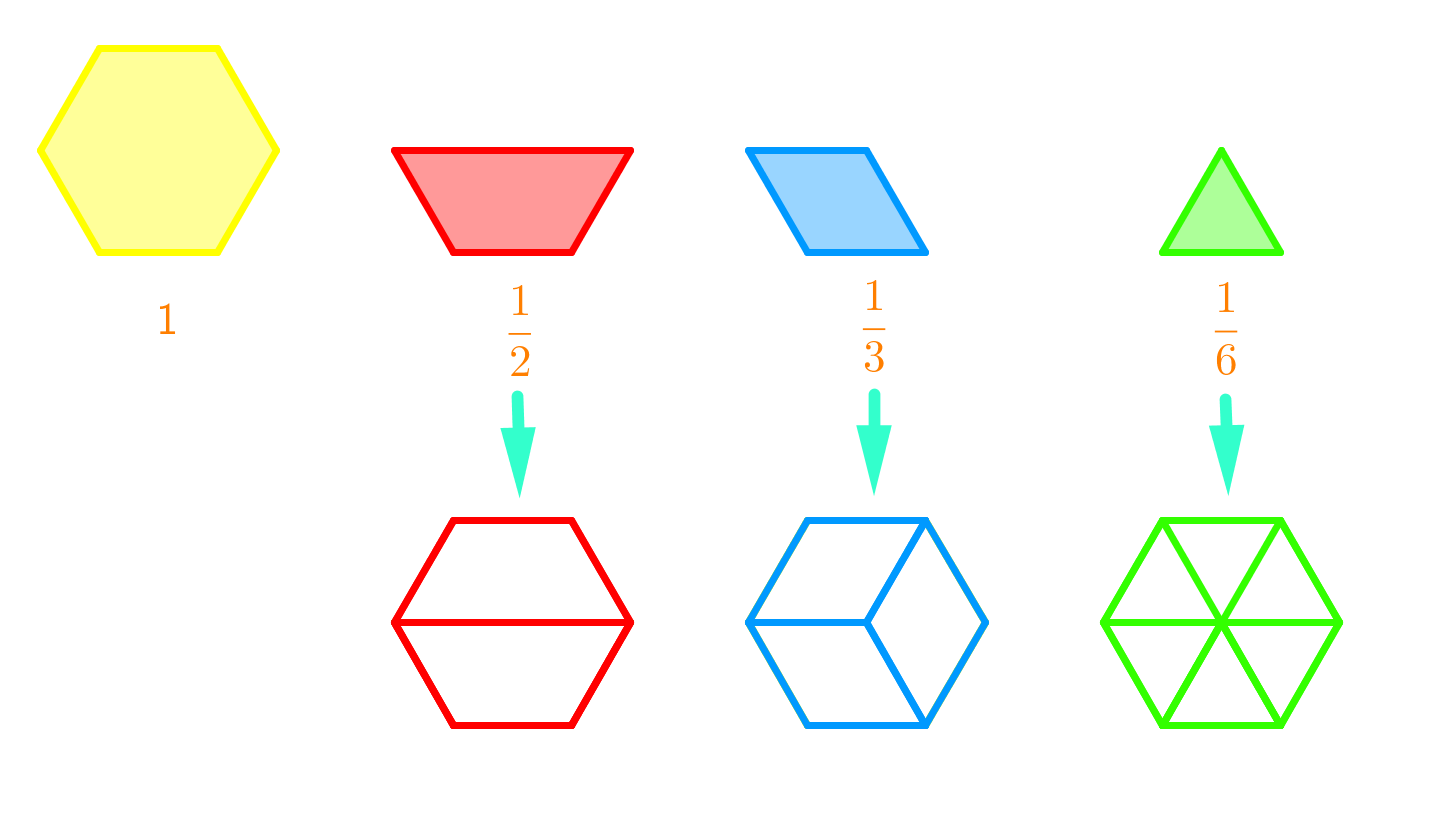1.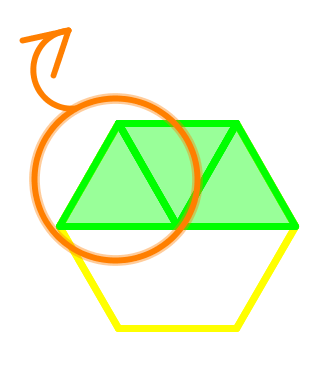2.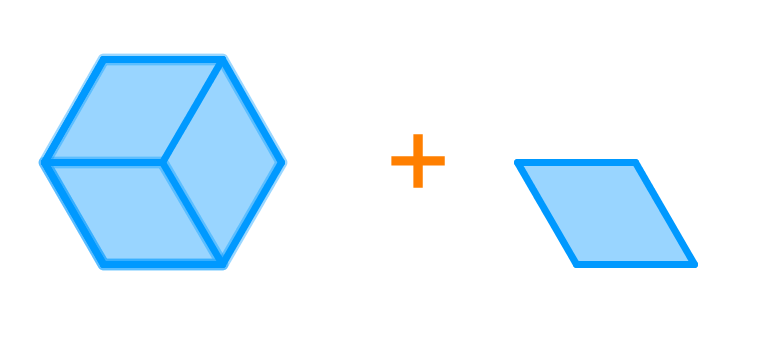3.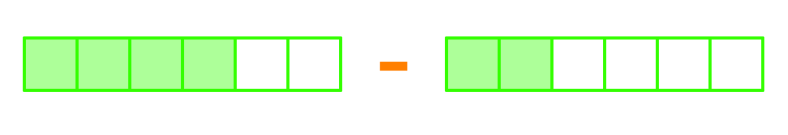4.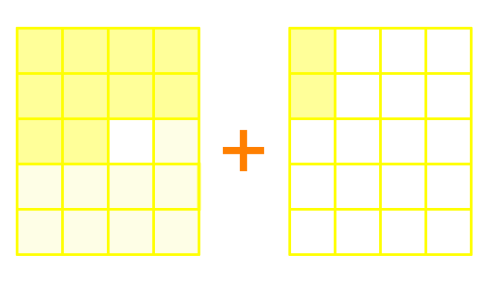2. Use a number line to calculate. Write the answer in lowest terms.
1. $\frac{5}{7}+\frac{1}{7}$
2. $\frac{2}{8}-\frac{1}{8}$
3. Each act in a play was $\frac{1}{2}$ hour long. There were 3 acts. How long was the play? Include a number line with your answer.
1. The diagram shows the layout of an aquatic centre. The pool took up $\frac{2}{8}$ of the aquatic centre and the stands took up $\frac{3}{8}$ of the aquatic centre. What is the difference between the amount of space taken up by the pool versus the stands?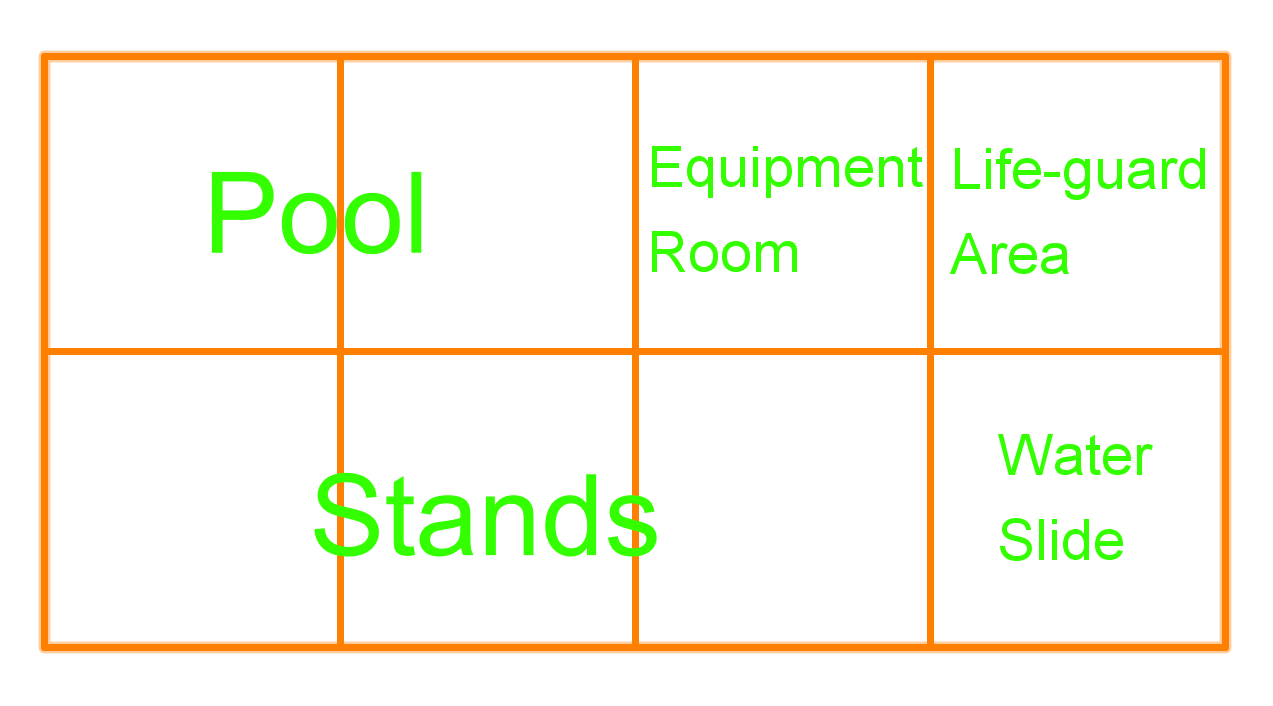0%
##### Practice
###### Free to Join!
StudyPug is a learning help platform covering math and science from grade 4 all the way to second year university. Our video tutorials, unlimited practice problems, and step-by-step explanations provide you or your child with all the help you need to master concepts. On top of that, it's fun - with achievements, customizable avatars, and awards to keep you motivated.
• #### Easily See Your ProgressWe track the progress you've made on a topic so you know what you've done. From the course view you can easily see what topics have what and the progress you've made on them. Fill the rings to completely master that section or mouse over the icon to see more details.
• #### Make Use of Our Learning Aids###### Practice Accuracy

Get quick access to the topic you're currently learning.

See how well your practice sessions are going over time.

Stay on track with our daily recommendations.

• #### Earn Achievements as You LearnMake the most of your time as you use StudyPug to help you achieve your goals. Earn fun little badges the more you watch, practice, and use our service.
• #### Create and Customize Your AvatarPlay with our fun little avatar builder to create and customize your own avatar on StudyPug. Choose your face, eye colour, hair colour and style, and background. Unlock more options the more you use StudyPug.
###### Topic Notes
In this section, we will write addition or subtraction statements involving fractions for given diagrams. We were introduced to addition and subtraction statements in previous sections. In this section, we will also be asked to use diagrams to solve word problems involving the addition and subtraction of fractions.

## How to add fractions

Before we begin this chapter, make sure you've got a good grip on prime factorization and divisibility rules.

When adding fractions, the first thing you'll have to do is make sure you have common denominators. This means that the number at the bottom of your fractions have to be the same. If your denominators aren't the same, you'll have to convert them so that they are.

You can get common denominators by taking the LCM (least common multiple) of the numbers in your denominator. Then you'll be able to add the numerators as you would with regular numbers when you carry out addition.

## How to subtract fractions

When subtracting fractions, you'll also have to make sure that the denominators are the same. After you've found the common factors for the denominators, you can once again carry out subtraction.

Let's try out practice problems to see how this works. We'll demonstrate the concept using models to add fractions and using models to subtract fractions. This means you'll learn how to visually add and subtract fractions.

## Example problems

Question 1:

Use a number line to calculate. Write the answer in lowest terms.

$\frac{5}{7} + \frac{1}{7}$

Solution:

So let's first draw the number line. Look at it and you see the number line from 0 to 1 is divided into 7 sections. This is because we are dealing with a denominator of 7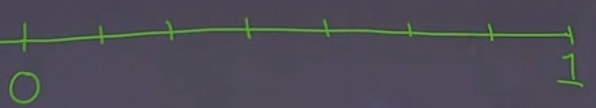Divide 1 into 7 intervals (denominator = 7)

If the denominator is 7, one way to represent 1 in fraction form is $\frac{7}{7}$ = 1. With the number line in 7 sections, we can calculate $\frac{5}{7} + \frac{1}{7}$ on the number line:

Look at $\frac{5}{7}$, it is on this location of the number line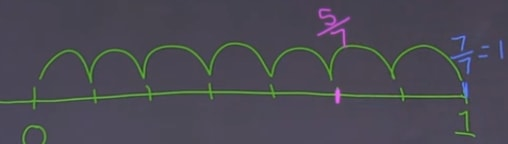Locate five seventh on the seven small segments

And we are adding $\frac{1}{7}$, and that's the result

And our final answer is $\frac{6}{7}$

Question 2:

Use a number line to calculate. Write the answer in lowest terms.

$\frac{2}{8} - \frac{1}{8}$

Solution:

So, let's draw the number line again. Look at it and you'll see the number line from 0 to 1 is divided into 8 sections. This is because we are dealing with a denominator of 8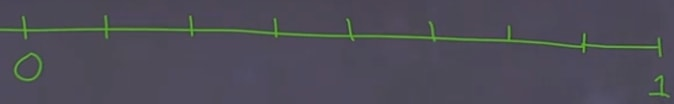Divide 1 into 8 segments (denominator = 8)

If the denominator is 8, one way to represent 1 in fraction form is $\frac{8}{8}$ = 1. With the number line in 8 sections, we can calculate $\frac{2}{8} - \frac{1}{8}$ on the number line:

Look at $\frac{2}{8}$, it is on this location of the number line

And we are subtracting $\frac{1}{8}$, and that's the result

And our answer is $\frac{1}{8}$

Question 3:

Write the addition or subtraction statement for the diagram. Write the answer in the lowest terms.

Solution:

We see the plus sign. So we are dealing with an addition. Each of the large rectangles represents a fraction. Each rectangle is divided into 20 sections. So 20 is the denominator. The shaded area represents the nominator.

So the first box:

It represents $\frac{10}{20}$

And the second box:

It represents $\frac{2}{20}$

So,

$\frac{10}{20} + \frac{2}{20} = \frac{12}{20}$

The question asks us to write the answer in the lowest terms. So, we need to look for the common factor of 12 and 20 in order to simplify the answer. Turns out 4 is the common factor. So, we'll divide 4 to the answer,

$\frac{12 \div 4}{20 \div 4} = \frac{3}{5}$

So

$\frac{3}{5}$

is the final answer.

Question 4:

Write the addition or subtraction statement for the diagram. Write the answer in the lowest terms.

Solution:

We see the minus sign. So we are dealing with a subtraction. Each of the rectangles represents a fraction. Each box is divided into 6 sections. So 6 is the denominator. The shaded area represents the nominator

So the first box:

It represents $\frac{4}{6}$

And the second box:

It represents $\frac{2}{6}$

So,

$\frac{4}{6} - \frac{2}{6} = \frac{2}{6}$

The question asks us to write the answer in the lowest terms. So, we need to look for the common factor of 2 and 6 in order to simplify the answer. Turns out 2 is the common factor. So, we divide 2 to the answer,

$\frac{2 \div 2}{6 \div 2} = \frac{1}{3}$

So

$\frac{1}{3}$

is the final answer.

Want to see an interactive diagram to see more of how to subtract fractions using a number line? Try this one out!

Next up, learn how to multiply whole numbers and fractions, and divide fractions with whole numbers. You can also learn how to multiply proper fractions.